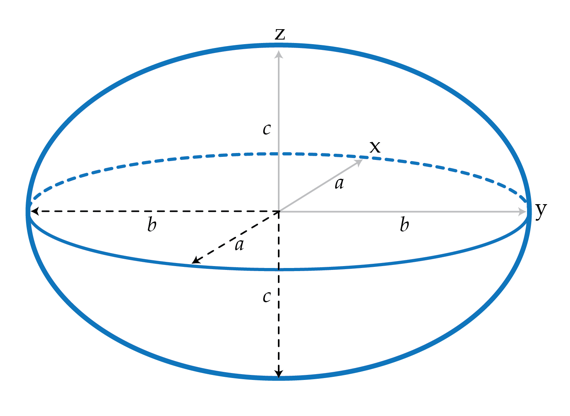# A charged ellipsoid

Determining how the charges distribute on the surface of a conductor is, in general, a very difficult problem. We know that if we charge a conductor the charges go to the surface and redistribute so that the electric field in the conductor vanishes. One of the few shapes for which this distribution can be determined analytically is the ellipsoid. ${\frac{x^{2}}{a^{2}} +\frac{y^{2}}{b^{2}}+ \frac{z^{2}}{c^{2}}}=1.$ Here, a, b and c are the ellipsoid's semi-axes. One can prove that for an ellipsoidal conductor the surface charge density is given by

$\sigma (x,y,z)=\frac{Q}{4\pi a b c}\frac{1}{\sqrt{ \frac{x^{2}}{a^{4}} +\frac{y^{2}}{b^{4}}+ \frac{z^{2}}{c^{4}}}}$ where Q is the the net charge of the conductor. Note that if we set $a=b=c=R$ we obtain the uniform charge distribution $\frac{Q}{4 \pi R^{2}}$, corresponding to a spherical conductor. Suppose that we measure the electric field near the surface of a charged ellipsoid with $Q=1nC$, $a=2 cm, b=5 cm$ and $c=3cm$. What is the maximum value in in volts per meter of the electric field?Details and assumptions

$k=\frac{1}{4\pi \epsilon_{0}}= 9\times 10^{9} m/F$

×

Problem Loading...

Note Loading...

Set Loading...